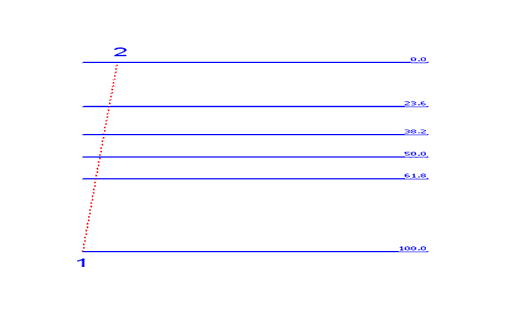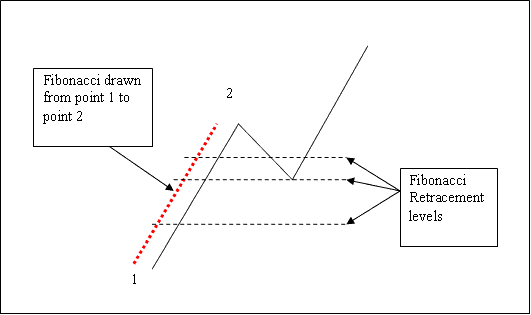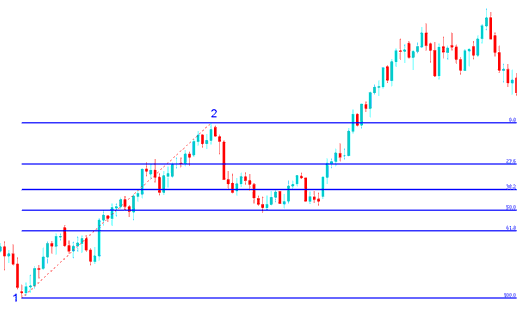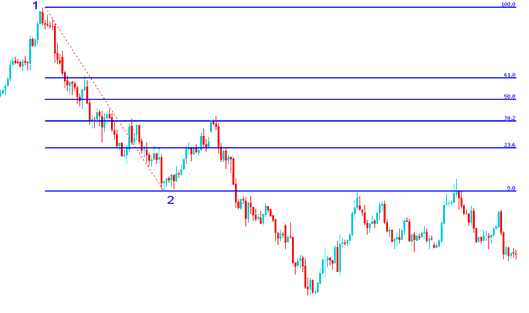# How To Use Indices Trading Fibonacci Retracement Levels

The indices trading Fibonacci retracement levels are explained below: traders should use this Indices Trading Fibonacci Retracement Levels stock indices indicator to determine where to open a trade whether a buy indices trade in a indices trading up indices trend and a sell indices trade in a downward indices trend.

How Do I Use Indices Trading Fibonacci Retracement Levels For Day Trading?How Do I Use Indices Trading Fibonacci Retracement Levels For Day Trading?

Indices Trading Fibonacci Retracement Tool ExplainedIndices Trading Fibonacci Retracement Tool Explained

## How Do I Use Indices Trading Fibonacci Retracement?

In the technical analysis example explained and illustrated below the stock indexes price is moving up between chart point 1 and chart point 2 then after chart point 2 it retraces down to 50.0% retracement level then stock indexes price continues moving up in the original upward indices trend. Note that this stock indices retracement indicator is drawn from point 1 to point 2 in the direction of the indices trend (Upward Direction).### Technical Analysis of How To Use Indices Trading Fibonacci Retracement in an Up Indices Trend

Once the stock indexes price hit the 50.0% retracement level, this retracement level provided a lot of support for the indices price, and afterwards the stock indexes trading market then resumed the original upward indices trend and continued to move up.

For this technical analysis example, the stock indexes price retracement reached the 50.0% retracement level, but most of the time the stock indexes trading market will retrace up to 38.2% retracement level and therefore most of the time indices traders set their buy limit indices orders at the 38.2% Fibonacci retracement level, while at the same time placing a stop just below 61.8% Fibonacci retracement level.

## How Do I Use Indices Trading Fibonacci Retracement?

In the Indices Trading Retracement Strategy example explained and illustrated below the stock indexes trading market is moving down between chart point 1 and chart point 2, then after chart point 2 the stock indexes price then retraces up to 38.2% retracement level then it continues moving downward in the original downward indices trend. Note that this stock indices retracement indicator is drawn from point 1 to point 2 in the direction of the indices trend (Downward Direction).### Technical Analysis of How To Use Indices Trading Fibonacci Retracement in a Down Indices Trend

The above technical analysis example is a stock indices retracement trading setup where the stock indexes price retraces immediately after touching the 38.2% Stock Indexes Chart Fibonacci Retracement Level.

In this technical analysis example the retracement of stock indexes price reached 38.2% retracement level and did not get to 50.0% retracement level. It is always good to use 38.2% retracement level because most times the stock indexes price retracement does not always get to 50.0% retracement level.

This Indices Trading Retracement level provided a lot of resistance for the stock indexes price retracement, this was the best place for a indices trader to place a sell limit indices order as the stock indexes trading market quickly moved down after hitting this stock indexes price retracement level.

Start Trading Crypto CFDs with a \$30 Bonus